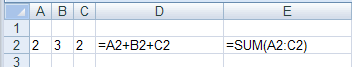# 6. Functions

 DEFINITION: A function is a standard routine used to perform common tasks.  It represents a complex formula that uses reserved words e.g. VLOOKUP, IF.

A function performs a specific set of operations on its input values to produce a single output value. The main spreadsheet applications available today have hundreds of ready-made functions that you can use.

For instance, one way to add up a list of items is to add them up one by one like thisThe formula in cell D2 can add up the three numbers in cells A2, B2, B3 but the function called SUM can also do the same thing. Creating a formula by hand to add up three numbers is very simple - but what if you had to add up 30,000 numbers? By far the easiest thing to do is to use the built-in function called SUM and provide it with the range of numbers to be added up.

This is the power of functions - they can carry out complicated operations on a set of numbers very easily. Other very common functions are

• VLOOKUP (looks up a value in a table)
• AVERAGE (works out the average of a set of numbers)
• COUNTIF (adds up numbers meeting a certain criteria)
• STDEV ( statistical function called standard deviation)
• DSUM (powerful way of summing values meeting a given criteria)
• WEEKNUM (finds the week number in the year that the input date falls within)

Functions can also be combined in a formula to make even more complicated operations. For example

=SUM(A1:A4) * AVERAGE(C2:C5) + VLOOKUP(A1,PRICE_LIST,2,FALSE)

This formula sums a range of values, multiplies the result by the average of another range of numbers then finally adds another number obtained from a look-up table.

People can be very creative in combining functions to work out complicated problems.

#### User defined functions

Although the ready-made functions are very handy, sometimes a set of input numbers have to be operated on in a very specific way in order to work out a value. Perhaps you need a function to work out the discount you can offer a customer depending on a number of factors stored in various cells.

So the main spreadsheet applications will allow you to define you own functions that you can then use in just the same way as the built-in ones.

challenge see if you can find out one extra fact on this topic that we haven't already told you